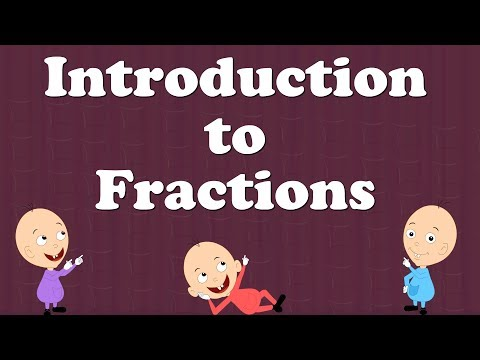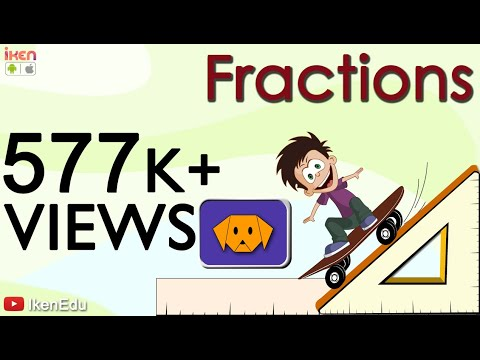## Fraction

Subject: Compulsory Maths

#### Overview

A fraction is a number which is usually expressed in the form of $\frac{a}{b}$. Multiplication of fraction can be done by the product of a whole number and a fraction as well as a product of a two fraction.

#### Fraction

A fraction is a number which is usually expressed in the form of $\frac{a}{b}$. For examples ( $\frac{3}{4}$, $\frac{5}{3}$, $\frac{9}{7}$, etc.)

Comparison of fraction

A fraction can be compared to their likes and unlike terms. We can compare like fractions by comparing their numerators and to compare unlike fractions, we should convert them into like fractions. By comparing those like fractions, as a result, we can find greater and the smaller fraction. For example, Let's compare $\frac{5}{3}$ and $\frac{7}{4}$

Here, the L.C.M of denominators 3 and 4 = 3×4 = 12

Now, $\frac{5}{3}$ = $\frac{5×4}{3×4}$ = $\frac{20}{12}$

And, $\frac{7×3}{4×3}$ = $\frac{21}{12}$

Here, $\frac{21}{12}$ > $\frac{20}{12}$, So, $\frac{7}{3}$ > $\frac{4}{3}$

If the like fraction is given, we can add or substract them just by adding or substracting their numenator. For example, $\frac{5}{6}$ + $\frac{7}{6}$ = $\frac{12}{6}$ = 2

$\frac{10}{7}$ - $\frac{9}{7}$ = $\frac{10 - 9}{7}$ = $\frac{1}{7}$ ans.

In case of unlike fraction, at first we should convert them into like fractions with the least common denominator, then we add or substract their numenator. For example,

or,$\frac{4}{5}$ - $\frac{3}{7}$ = $\frac{4 × 7}{5 × 7}$ - $\frac{3 × 5}{7 × 5}$

= $\frac{21}{35}$ - $\frac{15}{35}$

= $\frac{21 -15}{35}$

= $\frac{6}{35}$ ans.

Multiplication of fraction

Multiplication of fraction can be done by the product of a whole number and a fraction as well as product of a two fraction. For example 3× $\frac{4}{3}$

$\frac{3}{1}$ × $\frac{4}{3}$ = $\frac{12}{3}$ =4

$\frac{1}{3}$ × $\frac{6}{5}$ = $\frac{1×6}{3×5}$ = $\frac{6}{15}$ = $\frac{2}{5}$ ans.

Division of fraction

To divide a whole number by a fraction, we should multiply the whole number by the reciprocal of the fraction. For example,

4÷ $\frac{1}{6}$ = 4× $\frac{1}{6}$ = $\frac{4}{6}$ = $\frac{2}{3}$ ans.

##### Things to remember
• To divide a whole number by a fraction, we should multiply the whole number by the reciprocal of the fraction.
• By comparing those like fractions, as a result, we can find greater and the smaller fraction.
• It includes every relationship which established among the people.
• There can be more than one community in a society. Community smaller than society.
• It is a network of social relationships which cannot see or touched.
• common interests and common objectives are not necessary for society.
##### Videos for Fraction##### Introduction to Fractions for Kids##### Learn Fractions | Math Video To Learn Fraction And Its Uses##### Why Learn About Fractions and Decimals

soln:

2$\frac{3}{4}$+1$\frac{5}{8}$-3$\frac{1}{3}$

= $\frac{11}{4}$+$\frac{13}{8}$-$\frac{10}{3}$

=$\frac{11\times6+13\times3-10\times8}{24}$

=$\frac{66+39-80}{24}$

=$\frac{105-80}{24}$

=$\frac{25}{24}$

=1$\frac{1}{24}$

$\frac{2}{9}$+$\frac{4}{9}$

=$\frac{2+4}{9}$

=$\frac{6}{9}$

=$\frac{2}{3}$

soln:

$\frac{3}{5}$-$\frac{2}{7}$

=$\frac{3\times7-2\times5}{35}$

=$\frac{21-10}{35}$

=$\frac{11}{35}$

Soln:

$\frac{3}{5}$-$\frac{2}{7}$

=$\frac{3\times7}{5\times7}$ -$\frac{2\times5}{7\times5}$

=$\frac{21}{35}$ - $\frac{10}{35}$

=$\frac{21-10}{35}$

=$\frac{11}{35}$

Soln

$\frac{3}{5}$ of Rs 750=$\frac{3}{5}$ x Rs 750

=Rs450.

Solution:

Let the required sum be x.

Now, $\frac{2}{7}$ of x = Rs. 120

or, $\frac{2}{7}$ × x = Rs. 120

or, 2x = 7 × Rs. 120

or, x = $\frac{7 × Rs. 120}{2}$

= Rs. 420

So the required sum is Rs. 420.

Solution:

Let, he had x l of milk in the begining.

Remaining parts of the quantity of milk = 1 - $\frac{3}{4}$ = $\frac{1}{4}$ part.

Now, $\frac{1}{4}$ of x = 15 l

or, $\frac{1}{4}$ × x = 15 l

or, x = 4 × 15 l = 60 l

So, he had 60 l of milk in the begining.

Solution:

In 1 day, Kamala can do $\frac{1}{12}$ part of a piece of work.

In 3 days, Kamala can do 3 × $\frac{1}{12}$ part of work = $\frac{1}{4}$ parts of a piece of work

Now, the remaining parts of the work done by Reeta = (1-$\frac{1}{4}$) = $\frac{3}{4}$ parts.

So, $\frac{3}{4}$ parts of the work is done by Reeta.